# AY2021 June Revision J1 Revision Paper 1 Solutions1. (a)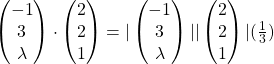1. (b)is parallel to, whereis a scalar.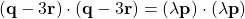2. Using partial fractions, we find thatUsing method of differences (DIY), we have that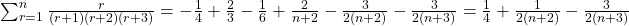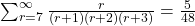3.differs fromby less than 1Plot the graph of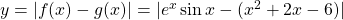andin the GC, sketch it out. * You can key in modulus by pressing (alpha)(window)(1).

Observe that the-coordinates of the intersections points between the graphs are..

4.Replacewith.5. (i).5. (ii) Let, then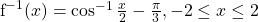.

5. (iii)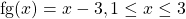6. (a)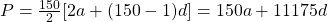Sum of first 40 even-numbered termsWe have6. (b)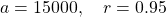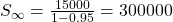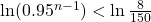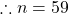years of extraction

7.

8. We substitute the given points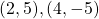andinto. We have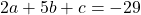—(1)—(2)—(3)

Using GC,We have.

We observe we have a circle with centreand radius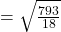9. (i) Equations of asymptotes:.

9. (ii) Maxand Min. Axial intercepts:and.

9. (iii)(a) From the sketch, the linedoes not cut the curve when.

(b) From the sketch, two real roots when.

10.Not readable? Change text.